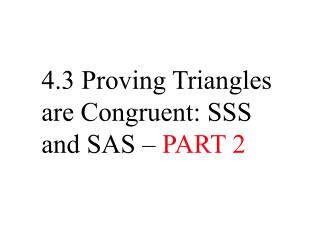# 4.3 Proving Triangles are Congruent: SSS and SAS – PART 2 - PowerPoint PPT PresentationDownload Presentation4.3 Proving Triangles are Congruent: SSS and SAS – PART 2

4.3 Proving Triangles are Congruent: SSS and SAS – PART 2
Download Presentation## 4.3 Proving Triangles are Congruent: SSS and SAS – PART 2

- - - - - - - - - - - - - - - - - - - - - - - - - - - E N D - - - - - - - - - - - - - - - - - - - - - - - - - - -
##### Presentation Transcript

1. 4.3 Proving Triangles are Congruent: SSS and SAS – PART 2

2. Congruent Triangles in a Coordinate Plane AC FH ABFG Use the SSS Congruence Postulate to show that ABCFGH. SOLUTION AC = 3 and FH= 3 AB = 5 and FG= 5

3. Congruent Triangles in a Coordinate Plane d = (x2 – x1 )2+ (y2 – y1 )2 d = (x2 – x1 )2+ (y2 – y1 )2 BC = (–4 – (–7))2+ (5– 0)2 GH = (6 – 1)2+ (5– 2)2 = 32+ 52 = 52+ 32 = 34 = 34 Use the distance formula to find lengths BC and GH.

4. Congruent Triangles in a Coordinate Plane BCGH BC = 34 and GH= 34 All three pairs of corresponding sides are congruent, ABCFGH by the SSS Congruence Postulate.

5. Congruent Triangles in a Coordinate Plane MN DE PMFE Use the SSS Congruence Postulate to show that NMPDEF. SOLUTION MN = 4 and DE= 4 PM = 5 and FE= 5

6. Congruent Triangles in a Coordinate Plane d = (x2 – x1 )2+ (y2 – y1 )2 d = (x2 – x1 )2+ (y2 – y1 )2 PN = (–1 – (– 5))2+ (6– 1)2 FD = (2 – 6)2+ (6– 1)2 = 42+ 52 = (-4)2+ 52 = 41 = 41 Use the distance formula to find lengths PN and FD.

7. Congruent Triangles in a Coordinate Plane PNFD PN = 41 and FD= 41 All three pairs of corresponding sides are congruent, NMPDEF by the SSS Congruence Postulate.

8. SAS  postulate SSS  postulate

9. T C S G The vertex of the included angle is the point in common. SSS  postulate SAS  postulate

10. SSS  postulate Not enough info

11. SSS  postulate SAS  postulate

12. Not Enough Info SAS  postulate

13. SSS  postulate Not Enough Info

14. SAS  postulate SAS  postulate